# Triangle

The essential made for every moment of your week—features transformable and adjustable straps for weightless support with no digging or tugging.

## Filters (0)

• Best Seller
• XS
• XS
• XS
• XS
• XS
• XS
• XS
• XS
• XS
• XS
• S
• S
• S
• S
• S
• S
• S
• S
• S
• S
• M
• M
• M
• M
• M
• M
• M
• M
• M
• M
• L
• L
• L
• L
• L
• L
• L
• L
• L
• L
• XL
• XL
• XL
• XL
• XL
• XL
• XL
• XL
• XL
• XL
• 2XL
• 2XL
• 2XL
• 2XL
• 2XL
• 2XL
• 2XL
• 2XL
• 2XL
• 2XL
• 3XL
• 3XL
• 3XL
• 3XL
• 3XL
• 3XL
• 3XL
• 3XL
• 3XL
• 3XL
• 1+
• 1+
• 1+
• 1+
• 1+
• 1+
• 1+
• 1+
• 1+
• 1+
• 2+
• 2+
• 2+
• 2+
• 2+
• 2+
• 2+
• 2+
• 2+
• 2+
• 3+
• 3+
• 3+
• 3+
• 3+
• 3+
• 3+
• 3+
• 3+
• 3+

Ultra-Soft Re:Play

1628

### Triangle Bralette

£21–£24

£14.70–£16.80

••••••••••• Best Seller
• XS
• XS
• XS
• XS
• XS
• XS
• XS
• XS
• XS
• XS
• S
• S
• S
• S
• S
• S
• S
• S
• S
• S
• M
• M
• M
• M
• M
• M
• M
• M
• M
• M
• L
• L
• L
• L
• L
• L
• L
• L
• L
• L
• XL
• XL
• XL
• XL
• XL
• XL
• XL
• XL
• XL
• XL
• 2XL
• 2XL
• 2XL
• 2XL
• 2XL
• 2XL
• 2XL
• 2XL
• 2XL
• 2XL
• 3XL
• 3XL
• 3XL
• 3XL
• 3XL
• 3XL
• 3XL
• 3XL
• 3XL
• 3XL
• 1+
• 1+
• 1+
• 1+
• 1+
• 1+
• 1+
• 1+
• 1+
• 1+
• 2+
• 2+
• 2+
• 2+
• 2+
• 2+
• 2+
• 2+
• 2+
• 2+
• 3+
• 3+
• 3+
• 3+
• 3+
• 3+
• 3+
• 3+
• 3+
• 3+

Sexy Silky Mesh

515

### Triangle Bralette

£24–£26

£16.80–£18.20

••••••••••• XS
• XS
• XS
• XS
• XS
• S
• S
• S
• S
• S
• M
• M
• M
• M
• M
• L
• L
• L
• L
• L
• XL
• XL
• XL
• XL
• XL
• 2XL
• 2XL
• 2XL
• 2XL
• 2XL
• 3XL
• 3XL
• 3XL
• 3XL
• 3XL
• 1+
• 1+
• 1+
• 1+
• 1+
• 2+
• 2+
• 2+
• 2+
• 2+
• 3+
• 3+
• 3+
• 3+
• 3+

New:Cotton

182

### Triangle Bralette

£21

£14.70

•••••• Selling Fast!
• XS
• XS
• XS
• XS
• XS
• XS
• XS
• S
• S
• S
• S
• S
• S
• S
• M
• M
• M
• M
• M
• M
• M
• L
• L
• L
• L
• L
• L
• L
• XL
• XL
• XL
• XL
• XL
• XL
• XL
• 2XL
• 2XL
• 2XL
• 2XL
• 2XL
• 2XL
• 2XL
• 3XL
• 3XL
• 3XL
• 3XL
• 3XL
• 3XL
• 3XL
• 1+
• 1+
• 1+
• 1+
• 1+
• 1+
• 1+
• 2+
• 2+
• 2+
• 2+
• 2+
• 2+
• 2+
• 3+
• 3+
• 3+
• 3+
• 3+
• 3+
• 3+

SuperSoft

176

### Triangle Bralette

£24

£16.80

•••••••• New!
• XS
• XS
• XS
• XS
• XS
• XS
• XS
• XS
• XS
• S
• S
• S
• S
• S
• S
• S
• S
• S
• M
• M
• M
• M
• M
• M
• M
• M
• M
• L
• L
• L
• L
• L
• L
• L
• L
• L
• XL
• XL
• XL
• XL
• XL
• XL
• XL
• XL
• XL
• 2XL
• 2XL
• 2XL
• 2XL
• 2XL
• 2XL
• 2XL
• 2XL
• 2XL
• 3XL
• 3XL
• 3XL
• 3XL
• 3XL
• 3XL
• 3XL
• 3XL
• 3XL
• 1+
• 1+
• 1+
• 1+
• 1+
• 1+
• 1+
• 1+
• 1+
• 2+
• 2+
• 2+
• 2+
• 2+
• 2+
• 2+
• 2+
• 2+
• 3+
• 3+
• 3+
• 3+
• 3+
• 3+
• 3+
• 3+
• 3+

Seamless Universal

2

### Triangle Bralette

£23

£16.10

•••••••••• New!
• XS
• XS
• XS
• XS
• S
• S
• S
• S
• M
• M
• M
• M
• L
• L
• L
• L
• XL
• XL
• XL
• XL
• 2XL
• 2XL
• 2XL
• 2XL
• 3XL
• 3XL
• 3XL
• 3XL
• 1+
• 1+
• 1+
• 1+
• 2+
• 2+
• 2+
• 2+
• 3+
• 3+
• 3+
• 3+

Ultra-Soft Re:Play

### Longline Triangle Bralette

£24

£16.80

••••• XS
• XS
• XS
• XS
• S
• S
• S
• S
• M
• M
• M
• M
• L
• L
• L
• L
• XL
• XL
• XL
• XL
• 2XL
• 2XL
• 2XL
• 2XL
• 3XL
• 3XL
• 3XL
• 3XL
• 1+
• 1+
• 1+
• 1+
• 2+
• 2+
• 2+
• 2+
• 3+
• 3+
• 3+
• 3+

Sexy Silky Mesh

### Triangle Bralette with Lace Trim

£27

£18.90

••••• XS
• XS
• XS
• XS
• S
• S
• S
• S
• M
• M
• M
• M
• L
• L
• L
• L
• XL
• XL
• XL
• XL
• 2XL
• 2XL
• 2XL
• 2XL
• 3XL
• 3XL
• 3XL
• 3XL
• 1+
• 1+
• 1+
• 1+
• 2+
• 2+
• 2+
• 2+
• 3+
• 3+
• 3+
• 3+

Sexy Silky Lace

74

### Triangle Bralette

£27

£18.90

•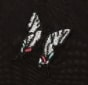••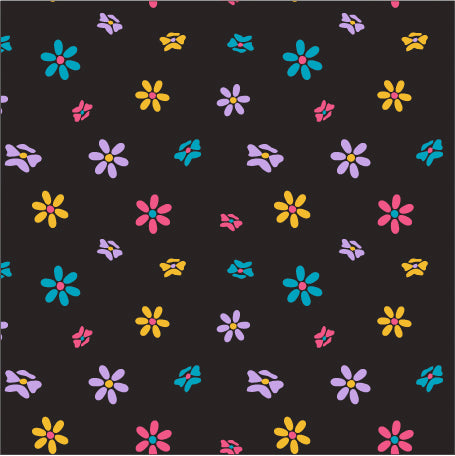•• XS
• XS
• XS
• XS
• XS
• XS
• XS
• XS
• S
• S
• S
• S
• S
• S
• S
• S
• M
• M
• M
• M
• M
• M
• M
• M
• L
• L
• L
• L
• L
• L
• L
• L
• XL
• XL
• XL
• XL
• XL
• XL
• XL
• XL
• 2XL
• 2XL
• 2XL
• 2XL
• 2XL
• 2XL
• 2XL
• 2XL
• 3XL
• 3XL
• 3XL
• 3XL
• 3XL
• 3XL
• 3XL
• 3XL
• 1+
• 1+
• 1+
• 1+
• 1+
• 1+
• 1+
• 1+
• 2+
• 2+
• 2+
• 2+
• 2+
• 2+
• 2+
• 2+
• 3+
• 3+
• 3+
• 3+
• 3+
• 3+
• 3+
• 3+

Lush Rib

17

### Triangle Bralette

£24

£16.80

••••••••• XS
• XS
• XS
• XS
• XS
• XS
• XS
• XS
• XS
• XS
• S
• S
• S
• S
• S
• S
• S
• S
• S
• S
• M
• M
• M
• M
• M
• M
• M
• M
• M
• M
• L
• L
• L
• L
• L
• L
• L
• L
• L
• L
• XL
• XL
• XL
• XL
• XL
• XL
• XL
• XL
• XL
• XL
• 2XL
• 2XL
• 2XL
• 2XL
• 2XL
• 2XL
• 2XL
• 2XL
• 2XL
• 2XL
• 3XL
• 3XL
• 3XL
• 3XL
• 3XL
• 3XL
• 3XL
• 3XL
• 3XL
• 3XL
• 1+
• 1+
• 1+
• 1+
• 1+
• 1+
• 1+
• 1+
• 1+
• 1+
• 2+
• 2+
• 2+
• 2+
• 2+
• 2+
• 2+
• 2+
• 2+
• 2+
• 3+
• 3+
• 3+
• 3+
• 3+
• 3+
• 3+
• 3+
• 3+
• 3+

Sexy Silky Mesh

37

### Strappy Triangle Bralette

£26–£29

£18.20–£20.30

•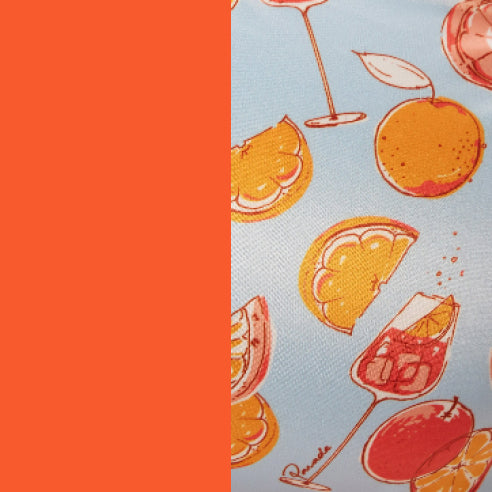••••••••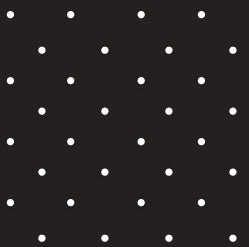•• XS
• XS
• XS
• XS
• XS
• XS
• XS
• XS
• XS
• XS
• S
• S
• S
• S
• S
• S
• S
• S
• S
• S
• M
• M
• M
• M
• M
• M
• M
• M
• M
• M
• L
• L
• L
• L
• L
• L
• L
• L
• L
• L
• XL
• XL
• XL
• XL
• XL
• XL
• XL
• XL
• XL
• XL
• 2XL
• 2XL
• 2XL
• 2XL
• 2XL
• 2XL
• 2XL
• 2XL
• 2XL
• 2XL
• 3XL
• 3XL
• 3XL
• 3XL
• 3XL
• 3XL
• 3XL
• 3XL
• 3XL
• 3XL
• 1+
• 1+
• 1+
• 1+
• 1+
• 1+
• 1+
• 1+
• 1+
• 1+
• 2+
• 2+
• 2+
• 2+
• 2+
• 2+
• 2+
• 2+
• 2+
• 2+
• 3+
• 3+
• 3+
• 3+
• 3+
• 3+
• 3+
• 3+
• 3+
• 3+

SuperSoft

34

### Strappy Bralette

£24–£26

£16.80–£18.20

••••••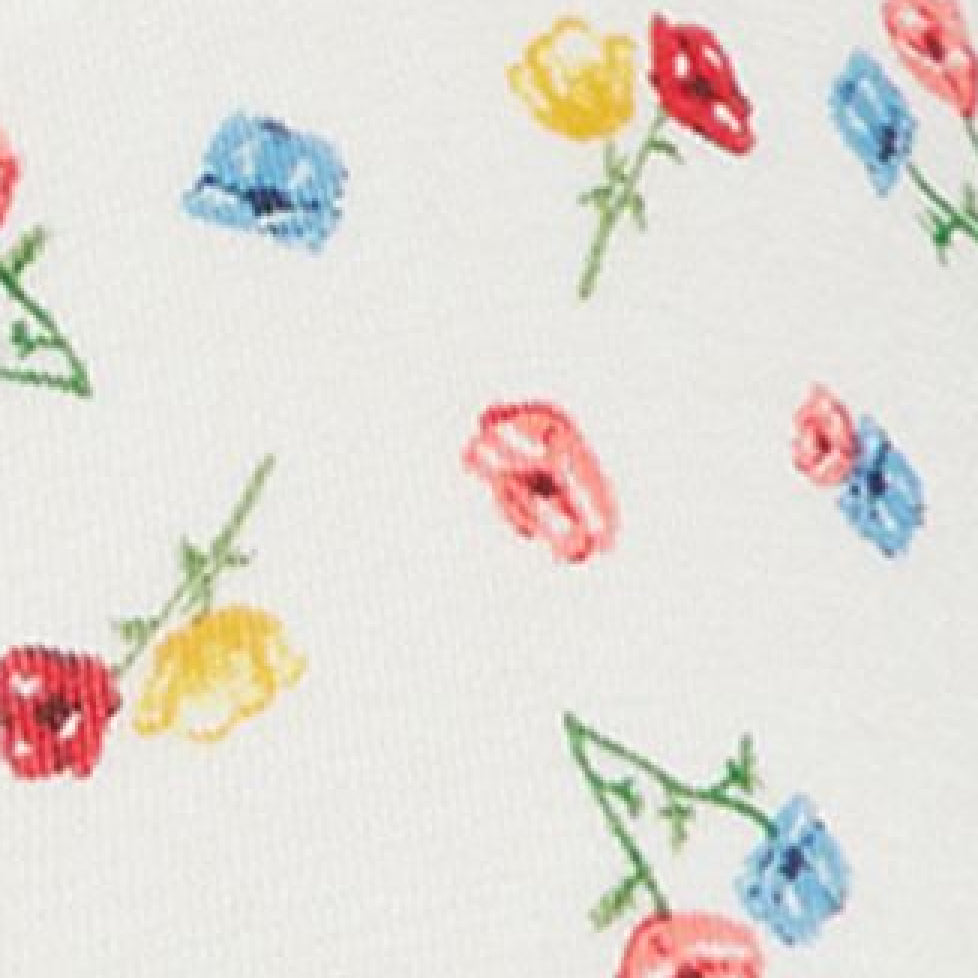••••• XS
• XS
• XS
• XS
• XS
• S
• S
• S
• S
• S
• M
• M
• M
• M
• M
• L
• L
• L
• L
• L
• XL
• XL
• XL
• XL
• XL
• 2XL
• 2XL
• 2XL
• 2XL
• 2XL
• 3XL
• 3XL
• 3XL
• 3XL
• 3XL
• 1+
• 1+
• 1+
• 1+
• 1+
• 2+
• 2+
• 2+
• 2+
• 2+
• 3+
• 3+
• 3+
• 3+
• 3+

Ultra-Soft Re:Play Naked

18

### Triangle Bralette

£21

£14.70

•••••• XS
• XS
• XS
• S
• S
• S
• M
• M
• M
• L
• L
• L
• XL
• XL
• XL
• 2XL
• 2XL
• 2XL
• 3XL
• 3XL
• 3XL
• 1+
• 1+
• 1+
• 2+
• 2+
• 2+
• 3+
• 3+
• 3+

Sexy Silky Mesh

40

### Triangle Bralette Hardware

£24

£16.80

•••• ### Re:Play Triangle Bralette

“This bralette is so comfy and sexy I did not want to take it off.”

Muriel W.